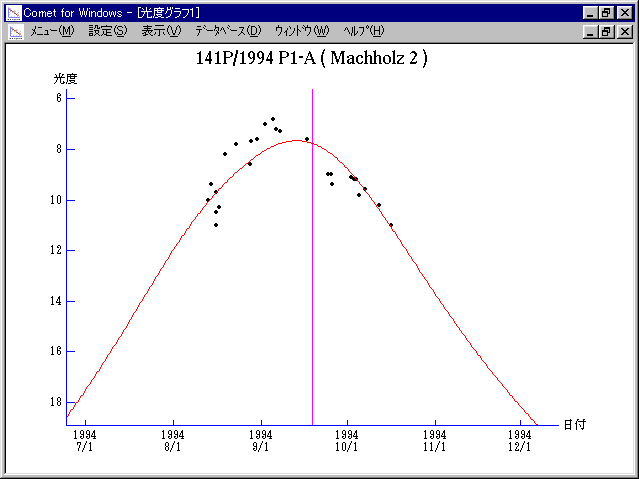# 141P/Machholz 2 (1994)###Profile

 Designation 141P/1994 P1 Recovery Date August 13, 1994 Magnitude 10 mag Recoverer Donald E. Machholz (Colfax, CA)

###Orbital Elements

```                    Epoch = 1994 Sept. 5.0 TT
T = 1994 Sept.18.8041 TT         Peri. = 149.2623
e = 0.750145                     Node  = 246.1772  2000.0
q = 0.752548 AU                  Incl. =  12.7865
a =  3.011944 AU    n = 0.1885532    P =   5.227 years
```

###Magnitudes Graph

```	Component A:
m1 = 13 + 5 log d + 35 log r

Component D:
m1 = 15.5 + 5 log d + 45 log r(t - 10)
```##### The orbital elements are calculated by Dr. Green and printed on IAUC 6108. The magnitudes graphs are made with Comet for Windows.